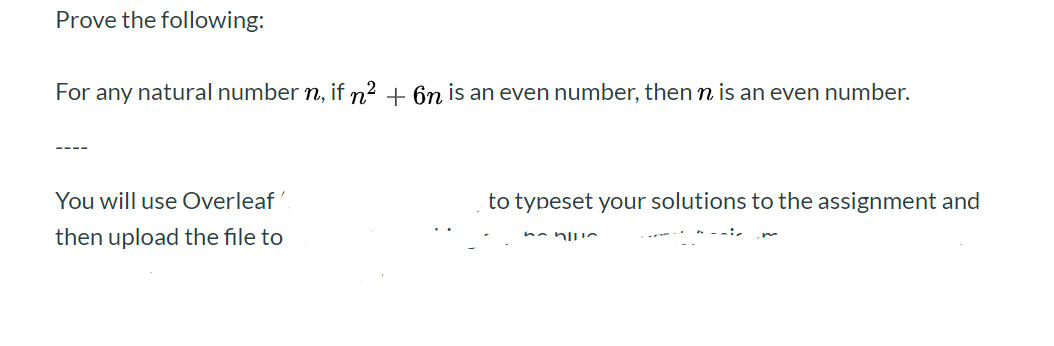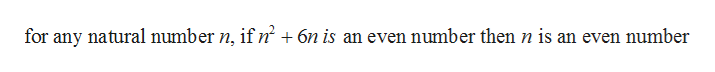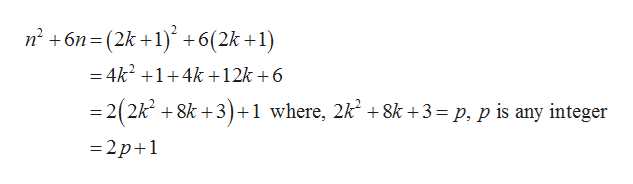# Prove the following:For any natural number n, if n2 6n is an even number, then n is an even number.-You will use Overleafto typeset your solutions to the assignment andthen upload the file to

Question
3 views

Define an integer k to be odd if k − 1 is even. Write up a formal proof of the following, using an indirect proof:

Claim: For any natural numbers m and n, if m is odd and n is odd, then m + n is even.help_outlineImage TranscriptioncloseProve the following: For any natural number n, if n2 6n is an even number, then n is an even number. - You will use Overleaf to typeset your solutions to the assignment and then upload the file to fullscreen
check_circle

Step 1

Consider the following information and prove the following:help_outlineImage Transcriptionclosefor any natural number n, if n' +6n is an even number then n is an even number fullscreen
Step 2

Prove the above result by contradiction:

Since, if n2+6n is an even number then n is an even number.

Let’s ...help_outlineImage Transcriptionclosen26n (2k 1) +6(2k +1) = 4k2 +1+4k +12k +6 =2(2k 8k +3) +1 where, 2k 8k+3= p, p is any integer =2p+1 fullscreen

### Want to see the full answer?

See Solution

#### Want to see this answer and more?

Solutions are written by subject experts who are available 24/7. Questions are typically answered within 1 hour.*

See Solution
*Response times may vary by subject and question.
Tagged in

### Math